## Example 26.19 Confirmatory Factor Analysis: Cognitive Abilities

In this example, cognitive abilities of 64 students from a middle school were measured. The fictitious data contain nine cognitive test scores. Three of the scores were for reading skills, three others were for math skills, and the remaining three were for writing skills. The covariance matrix for the nine variables was obtained. A confirmatory factor analysis with three factors was conducted. The following is the input data set:

```title "Confirmatory Factor Analysis Using the FACTOR Modeling Language";
title2 "Cognitive Data";
data cognitive1(type=cov);
_type_='cov';
writing1 writing2 writing3;
datalines;
reading1 83.024    .      .      .      .      .      .      .      .
reading2 50.924 108.243   .      .      .      .      .      .      .
reading3 62.205  72.050 99.341   .      .      .      .      .      .
math1    22.522  22.474 25.731 82.214   .      .      .      .      .
math2    14.157  22.487 18.334 64.423 96.125   .      .      .      .
math3    22.252  20.645 23.214 49.287 58.177 88.625   .      .      .
writing1 33.433  42.474 41.731 25.318 14.254 27.370 90.734   .      .
writing2 24.147  20.487 18.034 22.106 26.105 22.346 53.891 96.543   .
writing3 13.340  20.645 23.314 19.387 28.177 38.635 55.347 52.999 98.445
;
```

#### Confirmatory Factor Model with Uncorrelated Factors

You first fit a confirmatory factor model with uncorrelated factors to the data, as shown in the following statements:

```proc calis data=cognitive1 nobs=64 modification;
factor
Math_Factor   ---> math1-math3       ,
Write_Factor  ---> writing1-writing3 ;
pvar
Read_Factor Math_Factor Write_Factor = 3 * 1.;
cov
Read_Factor Math_Factor Write_Factor = 3 * 0.;
run;
```

In the PROC CALIS statement, the number of observations is specified with the NOBS= option. With the MODIFICATION in the PROC CALIS statement, LM (Lagrange Multiplier) tests are conducted. The results of LM tests can suggest the inclusion of additional parameters for a better model fit.

The FACTOR modeling language is most handy when you specify confirmatory factor models. You use the FACTOR statement to invoke the FACTOR modeling language. Entries in the FACTOR statement are for specifying factor-variables relationships and are separated by commas. In each entry, you first specify a latent factor, followed by the right arrow sign ---> (you can use >, ->, -->, or --->). Then you specify the observed variables that have nonzero loadings on the factor. For example, in the first entry of FACTOR statement, you specify that latent factor Read_Factor has nonzero loadings (free parameters) on variables reading1reading3. Optionally, you can specify the parameter list after you specify the factor-variable relationships. For example, you can name the loading parameters as in the following specification:

```factor
```

This way, you name the factor loadings with parameter names load1, load2, and load3, respectively. However, in the current example, because the loading parameters are all unconstrained, you can just let PROC CALIS to generate the parameter names for you. In this example, there are three factors: Read_Factor, Math_Factor, and Write_Factor. These factors have simple cluster structures with the nine observed variables. Each observed variable has only one loading on exactly one factor.

In the PVAR statement, you can specify the variances of the factors and the error variances of the observed variables. The factor variances in this model are all fixed at 1.0 for identification purposes. You do not need to specify the error variances of the observed variables in the current model because PROC CALIS assumes these are free parameters by default.

In the COV statement, you specify that the covariances among the factors are fixed zeros. There are three covariances among the three latent factors and therefore you put 3 * 0. for their fixed values. This means that the factors in the current model are uncorrelated. Note that you must specify uncorrelated factors explicitly in the COV statement because all latent factors are correlated by default.

In Output 26.19.1, the initial model specification is echoed in matrix form. The observed variables and factors are also displayed.

Output 26.19.1 Uncorrelated Factor Model Specification
Variables in the Model

 . [_Parm1]
 0
 0
 . [_Parm2]
 0
 0
 . [_Parm3]
 0
 0
math1
 0
 . [_Parm4]
 0
math2
 0
 . [_Parm5]
 0
math3
 0
 . [_Parm6]
 0
writing1
 0
 0
 . [_Parm7]
writing2
 0
 0
 . [_Parm8]
writing3
 0
 0
 . [_Parm9]

Initial Factor Covariance Matrix
Math_Factor 0 1.0000 0
Write_Factor 0 0 1.0000

Initial Error Variances
Variable Parameter Estimate

In the table for initial factor loading matrix, the nine loading parameters are shown to have simple cluster relations with the factors. In the table for initial factor covariance matrix, the diagonal matrix shows that the factors are not correlated. The diagonal elements are fixed at ones so that this matrix is also a correlation matrix for the factors. In the table for initial error variances, the nine variance parameters are shown. As described previously, these error variances are generated by PROC CALIS as default parameters.

In Output 26.19.2, initial estimates are generated by the instrumental variable method and the McDonald method.

Output 26.19.2 Optimization of the Uncorrelated Factor Model: Initial Estimates
Initial Estimation Methods
1 Instrumental Variables Method
2 McDonald Method

Optimization Start
Parameter Estimates
1 _Parm1 7.15372 0.00851
2 _Parm2 7.80225 -0.00170
3 _Parm3 8.70856 -0.00602
4 _Parm4 7.68637 0.00272
5 _Parm5 8.01765 -0.01096
6 _Parm6 7.05012 0.00932
7 _Parm7 8.76776 -0.0009955
8 _Parm8 5.96161 -0.01335
9 _Parm9 7.23168 0.01665

These initial estimates turn out to be pretty good, in the sense that only three more iterations are needed to converge to the maximum likelihood estimates and the final function value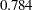does not change much from the initial function value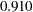, as shown in Output 26.19.3.

Output 26.19.3 Optimization of the Uncorrelated Factor Model: Iteration Summary
Iteration   Restarts Function
Calls
Active
Constraints
Objective
Function
Objective
Function
Change
Max Abs
Element
Lambda Ratio
Between
Actual
and
Predicted
Change
1   0 4 0   0.78792 0.1225 0.00175 0 0.932
2   0 6 0   0.78373 0.00419 0.000037 0 1.051
3   0 8 0   0.78373 5.087E-7 3.715E-9 0 1.001

Optimization Results
Iterations 3 Function Calls 11
Jacobian Calls 5 Active Constraints 0
Objective Function 0.783733415 Max Abs Gradient Element 3.7146571E-9
Lambda 0 Actual Over Pred Change 1.0006660673

 Convergence criterion (ABSGCONV=0.00001) satisfied.

The fit summary is shown in Output 26.19.4.

Output 26.19.4 Fit of the Uncorrelated Factor Model
Fit Summary
Modeling Info N Observations 64
N Variables 9
N Moments 45
N Parameters 18
N Active Constraints 0
Baseline Model Function Value 4.3182
Baseline Model Chi-Square 272.0467
Baseline Model Chi-Square DF 36
Pr > Baseline Model Chi-Square <.0001
Absolute Index Fit Function 0.7837
Chi-Square 49.3752
Chi-Square DF 27
Pr > Chi-Square 0.0054
Z-Test of Wilson & Hilferty 2.5474
Hoelter Critical N 52
Root Mean Square Residual (RMSR) 19.5739
Standardized RMSR (SRMSR) 0.2098
Goodness of Fit Index (GFI) 0.8555
Parsimony Index Adjusted GFI (AGFI) 0.7592
Parsimonious GFI 0.6416
RMSEA Estimate 0.1147
RMSEA Lower 90% Confidence Limit 0.0617
RMSEA Upper 90% Confidence Limit 0.1646
Probability of Close Fit 0.0271
ECVI Estimate 1.4630
ECVI Lower 90% Confidence Limit 1.2069
ECVI Upper 90% Confidence Limit 1.8687
Akaike Information Criterion 85.3752
Bozdogan CAIC 142.2351
Schwarz Bayesian Criterion 124.2351
McDonald Centrality 0.8396
Incremental Index Bentler Comparative Fit Index 0.9052
Bentler-Bonett NFI 0.8185
Bentler-Bonett Non-normed Index 0.8736
Bollen Normed Index Rho1 0.7580
Bollen Non-normed Index Delta2 0.9087
James et al. Parsimonious NFI 0.6139

Using the chi-square model test criterion, the uncorrelated factor model should be rejected at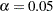. The RMSEA estimate is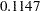, which is not indicative of a good fit according to Browne and Cudeck (1993) Other indices might suggest only a marginal good fit. For example, Bentler’s comparative fit index and Bollen nonnormed index delta2 are both above 0.90. However, many other do not attain this 0.90 level. For example, adjusted GFI is only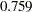. It is thus safe to conclude that there could be some improvements on the model fit.

The MODIFICATION option in the PROC CALIS statement has been used to request for computing the LM test indices for model modifications. The results are shown in Output 26.19.5.

Output 26.19.5 Lagrange Multiplier Tests
Variable Factor LM Stat Pr > ChiSq Parm
Change
math3 Write_Factor 3.58077 0.0585 1.89703
writing3 Math_Factor 1.87637 0.1707 1.41298
writing2 Math_Factor 0.86221 0.3531 0.95672
math1 Write_Factor 0.55602 0.4559 0.63906

Rank Order of the 3 Largest LM Stat for Covariances of Factors
Var1 Var2 LM Stat Pr > ChiSq Parm
Change
Write_Factor Math_Factor 7.07904 0.0078 0.40132

Rank Order of the 10 Largest LM Stat for Error Variances and Covariances
Error
of
Error
of
LM Stat Pr > ChiSq Parm
Change
writing1 math2 5.45986 0.0195 -13.16822
writing1 math1 5.05573 0.0245 12.32431
writing3 math3 3.93014 0.0474 13.59149
writing3 math1 2.83209 0.0924 -9.86342
writing2 math2 1.94879 0.1627 8.40273
writing2 math3 1.11704 0.2906 -7.23762

Three different tables for ranking the LM test results are shown. In the first table, the new loading parameters that would improve the model fit the most are shown first. For example, in the first row a new factor loading of writing1 on the Read_Factor is suggested to improve the model fit the most. The LM Stat value is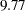. This is an approximation of the chi-square drop if this parameter was included in the model. The Pr > ChiSq value of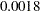indicates a significant improvement of model fit at. Nine more new loading parameters are suggested in the table, with less and less statistical significance in the change of model fit chi-square. Note that these approximate chi-squares are one-at-a-time chi-square changes. That means that the overall chi-square drop is not a simple sum of individual chi-square changes when you include two or more new parameters in the modified model.

The other two tables in Output 26.19.5 shows the new parameters in factor covariances, error variances, or error covariances that would result in a better model fit. The table for the new parameters of the factor covariance matrix indicates that adding each of the covariances among factors might lead to a statistically significant improvement in model fit. The largest LM Stat value in this table is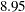, which is smaller than that of the largest LM Stat for the factor loading parameters. Despite this, it is more reasonable to add the covariance parameters among factors first to determine whether that improves the model fit.

#### Confirmatory Factor Model with Correlated Factors

To fit the corresponding confirmatory factor model with correlated factors, you can remove the fixed zeros from the COV statement in the preceding specification, as shown in the following statements:

```proc calis data=cognitive1 nobs=64 modification;
factor
Math_Factor   ---> math1-math3       ,
Write_Factor  ---> writing1-writing3 ;
pvar
Read_Factor Math_Factor Write_Factor = 3 * 1.;
cov
Read_Factor Math_Factor Write_Factor /* = 3 * 0. */;
run;
```

In the COV statement, you comment out the fixed zeros so that the covariances among the latent factors are now free parameters. An alternative way is to delete the entire COV statement so that the covariances among factors are free parameters by the FACTOR model default.

The fit summary of the correlated factor model is shown in Output 26.19.6.

Output 26.19.6 Fit of the Correlated Factor Model
Fit Summary
Modeling Info N Observations 64
N Variables 9
N Moments 45
N Parameters 21
N Active Constraints 0
Baseline Model Function Value 4.3182
Baseline Model Chi-Square 272.0467
Baseline Model Chi-Square DF 36
Pr > Baseline Model Chi-Square <.0001
Absolute Index Fit Function 0.4677
Chi-Square 29.4667
Chi-Square DF 24
Pr > Chi-Square 0.2031
Z-Test of Wilson & Hilferty 0.8320
Hoelter Critical N 78
Root Mean Square Residual (RMSR) 5.7038
Standardized RMSR (SRMSR) 0.0607
Goodness of Fit Index (GFI) 0.9109
Parsimony Index Adjusted GFI (AGFI) 0.8330
Parsimonious GFI 0.6073
RMSEA Estimate 0.0601
RMSEA Lower 90% Confidence Limit 0.0000
RMSEA Upper 90% Confidence Limit 0.1244
Probability of Close Fit 0.3814
ECVI Estimate 1.2602
ECVI Lower 90% Confidence Limit 1.2453
ECVI Upper 90% Confidence Limit 1.5637
Akaike Information Criterion 71.4667
Bozdogan CAIC 137.8032
Schwarz Bayesian Criterion 116.8032
McDonald Centrality 0.9582
Incremental Index Bentler Comparative Fit Index 0.9768
Bentler-Bonett NFI 0.8917
Bentler-Bonett Non-normed Index 0.9653
Bollen Normed Index Rho1 0.8375
Bollen Non-normed Index Delta2 0.9780
James et al. Parsimonious NFI 0.5945

The model fit chi-square value is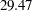, which is about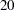less than the model with uncorrelated factors. The-value is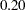, indicating a satisfactory model fit. The RMSEA value is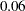, which is close to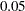, a value recommended as an indication of good model fit by Browne and Cudeck (1993) More fit indices that do not attain the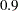level with the uncorrelated factor model now have values close to or above. These include the goodness-of-fit index (GFI), McDonald centrality, Bentler-Bonnet NFI, and Bentler-Bonnet nonnormed index. By all counts, the correlated factor model is a much better fit than the uncorrelated factor model.

In Output 26.19.7, the estimation results for factor loadings are shown. All these loadings are statistically significant, indicating non-chance relationships with the factors.

 6.7657 1.0459 6.4689 [_Parm01]
 0
 0
 7.8579 1.1890 6.6090 [_Parm02]
 0
 0
 9.1344 1.0712 8.5269 [_Parm03]
 0
 0
math1
 0
 7.5488 1.0128 7.4536 [_Parm04]
 0
math2
 0
 8.4401 1.0838 7.7874 [_Parm05]
 0
math3
 0
 6.8194 1.0910 6.2506 [_Parm06]
 0
writing1
 0
 0
 7.9677 1.1254 7.0797 [_Parm07]
writing2
 0
 0
 6.8742 1.1986 5.7350 [_Parm08]
writing3
 0
 0
 7.0949 1.2057 5.8844 [_Parm09]

In Output 26.19.8, the factor covariance matrix is shown. Because the diagonal elements are all ones, the off-diagonal elements are correlations among factors. The correlations range from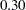. These factors are moderately correlated.

Output 26.19.8 Estimation of the Correlations of Factors
Factor Covariance Matrix: Estimate/StdErr/t-value
 1.0000
 0.3272 0.1311 2.4955 [_Parm10]
 0.4810 0.1208 3.9813 [_Parm11]
Math_Factor
 0.3272 0.1311 2.4955 [_Parm10]
 1.0000
 0.3992 0.1313 3.0417 [_Parm12]
Write_Factor
 0.4810 0.1208 3.9813 [_Parm11]
 0.3992 0.1313 3.0417 [_Parm12]
 1.0000

In Output 26.19.9, the error variances for variables are shown.

Output 26.19.9 Estimation of the Error Variances
Error Variances
Variable Parameter Estimate Standard
Error
t Value

Allvalues except the one for reading3 are greater than, a value close to a criticalvalue at. This means that the error variance for reading3 could have been zero in the population, or it could have been nonzero but the current sample just has this insignificant value by chance (that is, a Type 2 error). Further research is needed to confirm either way.

In addition to the parameter estimation results, PROC CALIS also outputs supplementary results that could be useful for interpretations. In Output 26.19.10, the squared multiple correlations and the factor scores regression coefficients are shown.

Output 26.19.10 Supplementary Estimation Results
Squared Multiple Correlations
Variable Error Variance Total Variance R-Square
math1 25.22889 82.21400 0.6931
math2 24.89032 96.12500 0.7411
math3 42.12110 88.62500 0.5247
writing1 27.24965 90.73400 0.6997
writing2 49.28881 96.54300 0.4895
writing3 48.10684 98.44500 0.5113

Factor Scores Regression Coefficients
The percentages of variance for the observed variables that can be explained by the factors are shown in the R-Square column of the table for squared multiple correlations (R-squares). These R-squares can be interpreted meaningfully because there is no reciprocal relationships among variables or correlated errors in the model. All estimates of R-squares are bounded betweenand.